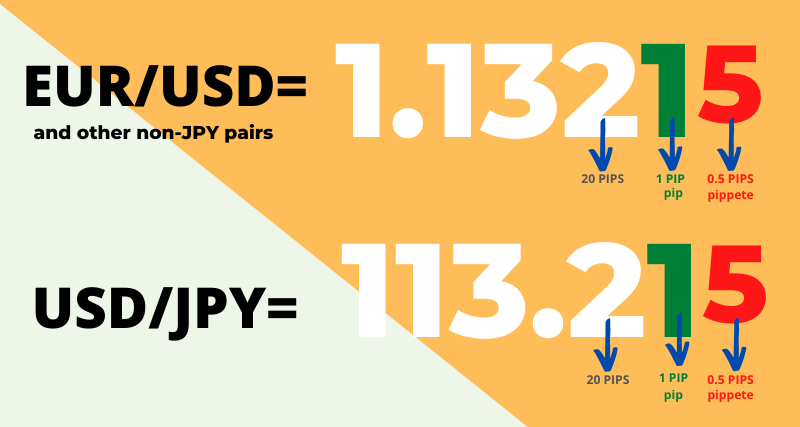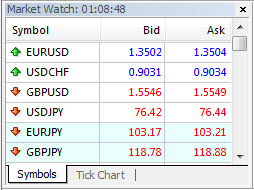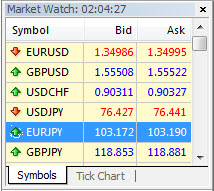When you are into weightlifting, the common lingo of performance is pounds: “I bench pressed 150 pounds today.” When you are into forex, the common lingo of performance is pips: “I made 150 pips profit on my last trade” or “My trade was stopped out today and I lost 85 pips.”

## What is a Pip in Forex Trading

The term Pip is the short abbreviation for “percentage in point” or “price interest point” and is the smallest incremental price move of a currency pair. It’s the last decimal point in exchange rates or currency pairs. Depending on context, this is normally one basis point 0.0001 in the case of EUR/USD, GBD/USD, USD/CHF and .01 in the case of USD/JPY. It is easy to see with examples.

Here is a 4-digit broker example:Note: With 4-digit brokers, this last decimal point is 0.0001 for most currency pairs, and 0.01 for Yen based currency pairs. The spread is 2 pips (1.3504-1.3502=2) on this Admiral Markets demo. If the EUR/USD moves from 1.3502 to 1.3503, it would have moved ONE PIP.

 Entry Long EUR/USD at 1.3505 Exit Close EUR/USD at 1.3525 Profit/Loss +20 Pips

If you buy EUR/USD at 1.3505 and sell at 1.3525, you would have made 20 pips.

 Entry Short USD/JPY at 88.95 Exit Close USD/JPY at 89.25 Profit/Loss -30 Pips

If you sold USD/JPY at 88.95 and exited at 89.25, you would have lost -30 pips.

Here is a 5-digit broker example:With 5-digit brokers (also called fractional pricing brokers), the last decimal point is 0.00001, or 0.001 for Yen based currencies, and it represents a fraction of a pip. For example, the spread on the EUR/USD is 0.9 pips (1.34995-1.34986) on this Alpari UK demo. If the EUR/USD moves from 1.34986 to 1.34996, it would have moved 1 pip.

 Entry Long GBP/USD at 1.55508 Exit Close GBP/USD at 1.56791 Profit/Loss +128.30 Pips

If you bought the GBP/USD at 1.55508 and sold it at 1.56791, you would have made 128.30 pips (1.56791-1.55508=128.30).

Because of the extra digit, it is harder to eyeball an exact pip spread or pip profit without the aid of a calculator. But this eyeballing problem of 5-digit brokers is outweighed by the larger advantage of typically better spreads, i.e., lower transaction costs, compared to their 4-digit broker counterparts.

The reason pips are so important is because they are the basis for calculating the dollar profit or loss in forex trading, as we shall see in the calculation of pip value (automatic and manual).

## What is the Difference Between a Pip, Tick and Tick Size

In Forex, the tick size and pip are almost synonymous. As we have seen, pip is the smallest incremental price move of a currency. Tick size is the smallest possible change in price – very similar. Just like futures, the minimum possible amount that a price can move is also the market’s tick size. With 4-digit brokers, the EUR/USD has a tick size of 0.0001, which means that the smallest increment that the price can move from 1.3840 would be up to 1.3841 or down to 1.3839.

The EUR futures market also has a tick size of 0.0001, but the difference is that the tick size of one contract has a fixed tick value of \$12.50, meaning that for every 0.0001 that the price moves up or down, the profit or loss of the trade would increase or decrease by \$12.50. In contrast, the tick size (pip or fraction of pip) of forex pairs is not fixed to a dollar value, and is instead calculated relative to the units traded (for Direct Rates), and also to the exchange rate and base currency quote (for Indirect Rates and Cross Rates).

## How to Calculate Pip Value and Profit/Loss Manually

Bear in mind that the calculation of the dollar pip value is done by the broker automatically. However, for a number of reasons, it is useful to know how to do it on your own.

The calculation of profit/loss is different depending on the currency rate types, and there are three of them:

Rate TypesCurrency Pairs
Direct RatesEUR/USD, GBP/USD, AUD/USD, NZD/USD
Cross RatesEUR/JPY, GBP/JPY, AUD/JPY, EUR/CHF, EUR/GBP

There is a way to calculate the pip value of each pair and multiply it with the pip movement to derive the profit and loss in dollars. If you don’t want to do pip value calculations, you can always use a handy indicator that displays the pip value (along with bid, spread, buy/sell swap rate) of a multiplicity of pairs: Display Info All Pairs, by Hanover. This indicator will display the following info, for all currency pairs offered by your broker:

• Symbol (A=AUD, C=CAD, E=EUR, F=CHF, G=GBP, J=JPY, N=NZD, U=USD)
• Current bid price
• Daily range to date: ave daily range (DR as a % of ADR)
• Dollars per pip (per full lot traded)
• Swap paid(+) or charged(-) by broker on long and short positions

A picture of both this handy indicator applied to the AUDUSD chart of FXPro looks like this:

The dollar per pip value appears as third from the last column. UJ or USDJPY has a pip value of 12.44 (one of the bigger ones), and AN or AUDNZD has a pip value of 7.65 (one of the smaller ones). Notice that the AUDNZD spread is one of the largest at 20 pips (4th from the last column), which is a whopping 28.9% of average daily range: as soon you go long on this pair with a 100K lot, you would be down -\$153 (20 pips X 7.65). Not good.

Thankfully, most pairs have a spread less than 5 pips and a pip value close to 10. Going long EU or EURUSD would incur a spread cost of only 1.8 pips (2.8% of ADR) and at a pip value of \$10, you would be only down \$18 on a 100K lot. This is a sizable difference from the cost of trading AUDNZD. Below are ways to calculate pip values and pip movements through various calculations.

## Pip Value on Direct Rates

Direct rates are currency pairs where the USD is the quote currency (the second currency being quoted i.e. XXX/USD). The common base currency (first currency quoted) pairings include EUR, GBP, AUD, and NZD.

Below is a table illustrating how to derive the Pip Value, Profit / Loss (P/L) in Pips, and the P/L in \$USD.

Rate TypesFormulaExample: EUR/USD 200,000 contract
buy at 1.4204 and sold at 1.4378
Pip Valuelot size * tick size200,000 (lot size) * 0.0001 (tick size) = \$20.00
P/L in PipsSelling Price – Purchase Price1.4378 (selling price) – 1.4204 (purchase price) = .0174 positive pip difference = 174 pips profit
P/L in \$USDPip Profit (loss) * Pip Value174 (pip profit) * \$20 (pip value) = \$3480 USD

Note on Direct Rates:

Direct rates provide the easiest formula for calculating the pip value (lot size * tick size). Because the pip value does not divide with the current rate, as in the two rate formulas below, it remains the same value between the direct rate pairs and each pair’s price fluctuations. You can always remember that the pip value of a micro lot (1000 units) is \$0.1 (10 cents), that the pip value of a mini lot (10,000 units) is \$1, and that the pip value of a standard lot (100,000 units) is \$10.

## Pip Value on Indirect Rates

Indirect rates are those currencies traded indirectly against the U.S. Dollar (USD). For example, with USD/CAD, the USD is the “base currency,” the CAD is the “quote currency” and the rate quote is expressed as units per USD. USD/CAD trading at 0.9874 means that 1 USD = 0.9874.

Rate TypesFormulaExample: USD / JPY 100,000 contract
sell at 82.12 and buy at 81.34
Pip Valuelot size * tick size / current rate100,000 (lot size) * 0.01 (tick size)  / 82.12 (current rate) = \$12.17
P/L in PipsSelling Price – Purchase Price82.12 (selling price) – 81.34 (purchase price) = .78 positive pip difference = 78 pips
P/L in \$USDPip Profit (loss) * Pip Value78 (pip profit) * \$12.17 (pip value) = \$949 USD

Note on Indirect Rates:

The only difference in pip value calculation between indirect rates and direct rates is the division with the current rate (lot size * tick size/currency rate). This division takes away from the more fixed rate of the direct rates. It makes all the pip values between the indirect rate pairs differ, and all the pip values will dynamically change relative to the current rate that changes constantly.

## Pip Value on Cross Rates

Cross Rates are currency pairs that do not involve the USD. Interestingly enough, though the USD is not represented in the quote, it is used in the quote calculation. An example of a cross rate is the EUR/CHF, where EUR is the base currency and CHF is the quote currency.

Rate TypesFormulaExample: EUR/CHF 100,000 contract
buy at  1.1500 and sell at 1.1620
(with EUR/USD at 1.3890)
Pip Valuelot size * tick size * base quote / current rate100,000 (lot size) * 0.0001 (tick size)  * 1.3890 (EUR/USD base quote) / 1.1500 (current rate) = \$12.07
P/L in PipsSelling Price – Purchase Price1.1620 (selling price) – 1.1500 (purchase price) = 0.0120 positive pip difference = 120 pips
P/L in \$USDPip Profit (loss) * Pip Value120 (pip profit) * \$12.07 (pip value) = \$1448 USD

Note on Cross Rates:

The difference between this formula and the indirect one is that in the calculation of the pip value there is an extra multiple with the base quote, which in this example above is the current EUR/USD price. This makes the calculation a little more involved than the previous two. These extra calculations with base quote and division with current rate makes all the pip values between the cross rate pairs differ, and all the pip values will dynamically change relative to the current rate that itself changes constantly.

### Here is a table breakdown of all the formulas, side by side:

Rate TypesPip Value CalculationP/L CalculationP/L to USD Calculation
Direct RatesPip Value = lot size * tick sizeP/L = Selling Price – Purchase PriceUSD Profit = Pip Profit (loss) * Pip Value
Indirect RatesPip Value = lot size * tick size / current rateP/L = Selling Price – Purchase PriceUSD Profit= Pip Profit (loss) * lot size * Pip Value
Cross RatesPip Value = lot size * tick size * base quote / current rateP/L = Selling Price – Purchase PriceUSD Profit= Pip Profit (loss) * Pip Value

Handy Tip:

Do you need to memorise all this information to calculate the pip value? Surely not. There's a much easier way to do it. To easily calculate pip values, with precision and per currency pair use our handy tool:

Cashback Forex - Forex Pip Calculator